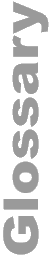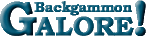EquityEquity The value of a position to one of the players. Equity is the sum of the values of the possible outcomes from a given position with each value multiplied by its probability of occurrence. It is the same as the fair settlement value of the position. Your equity is the negative of your opponent's equity. See post by Gary Wong. Equity comes in different flavors.  See: Cubeless Equity, Cubeful Equity, Match Equity, and EMG Equity.

 Help   •   About the Glossary   •   List of Terms   •   Full Glossary